# Variational Autoencoder with Tensorflow – X – VAE application to CelebA images

I continue with my series on Variational Autoencoders and methods to control the Kullback-Leibler [KL] loss.

The last method discussed made use of Tensorflow’s GradientTape()-class. We still have to test this approach on a challenging dataset like CelebA. Our ultimate objective will be to pick up randomly chosen data points in the VAE’s latent space and create yet unseen but realistic face images by the trained Decoder’s abilities. This task falls into the category of Generative Deep Learning. It has nothing to do with classification or a simple reconstruction of images. Instead we let a trained Artificial Neural Network create something new.

The code fragments discussed in the last post of this series helped us to prepare images of CelebA for training purposes. We cut and downsized them. We saved them in their final form in Numpy arrays: Loading e.g. 170,000 training images from a SSD as a Numpy array is a matter of a few seconds. We also learned how to prepare a Keras ImageDataGenerator object to create a flow of batches with image data to the GPU.

We have also developed two Python classes “MyVariationalAutoencoder” and “VAE” for the setup of a CNN-based VAE. These classes allow us to control a VAE’s input parameters, its layer structure based on Conv2D- and Conv2DTranspose layers, and the handling of the Kullback-Leibler [KL-] loss. In this post I will give you Jupyter code fragments that will help you to apply these classes in combination with CelebA data.

# Basic structure of the CNN-based VAE – and sizing of the KL-loss contribution

The Encoder and Decoder CNNs of our VAE shall consist of 4 convolutional layers and 4 transpose convolutional layers, respectively. We control the KL loss by invoking GradientTape() and train_step().

Regarding the size of the KL-loss:
Due to the “curse of dimensionality” we will have to choose the KL-loss contribution to the total loss large enough. We control the relative size of the KL-loss in comparison to the standard reconstruction loss by a parameter “fact“. To determine an optimal value requires some experiments. It also depends on the kind of reconstruction loss: Below I assume that we use a “Binary Crossentropy” loss. Then we must choose fact > 3.0 to get the KL-loss to become bigger than 3% of the total loss. Otherwise the confining and smoothing effect of the KL-loss on the data distribution in the latent space will not be big enough to force the VAE to learn general and not specific features of the training images.

# Imports and GPU usage

Below I present Jupyter cells for required imports and GPU preparation without many comments. Its all standard. I keep the Python file with the named classes in a folder “my_AE_code.models”. This folder must have been declared as part of the module search path “sys.path”.

# Jupyter Cell 1 – Imports

```import os, sys, time, random
import math
import numpy as np

import matplotlib as mpl
from matplotlib import pyplot as plt
from matplotlib.colors import ListedColormap
import matplotlib.patches as mpat

import PIL as PIL
from PIL import Image
from PIL import ImageFilter

# temsorflow and keras
import tensorflow as tf
from tensorflow import keras as K
from tensorflow.keras import backend as B
from tensorflow.keras.models import Model
from tensorflow.keras import regularizers
from tensorflow.keras import optimizers
from tensorflow.keras import metrics
from tensorflow.keras.layers import Input, Conv2D, Flatten, Dense, Conv2DTranspose, Reshape, Lambda, \
Activation, BatchNormalization, ReLU, LeakyReLU, ELU, Dropout, \

#from tensorflow.keras.utils import to_categorical
#from tensorflow.keras.optimizers import schedules

from tensorflow.keras.preprocessing.image import ImageDataGenerator

from my_AE_code.models.MyVAE_3 import MyVariationalAutoencoder
from my_AE_code.models.MyVAE_3 import VAE
```

Jupyter Cell 2 – List available Cuda devices

```# List Cuda devices
# Suppress some TF2 warnings on negative NUMA node number
# see https://www.programmerall.com/article/89182120793/
os.environ['TF_CPP_MIN_LOG_LEVEL'] = '3'  # or any {'0', '1', '2'}

tf.config.experimental.list_physical_devices()
```

Jupyter Cell 3 – Use GPU and limit VRAM usage

```# Restrict to GPU and activate jit to accelerate
# *************************************************
# NOTE: To change any of the following values you MUST restart the notebook kernel !

b_tf_CPU_only      = False   # we need to work on a GPU
tf_limit_CPU_cores = 4
tf_limit_GPU_RAM   = 2048

b_experiment  = False # Use only if you want to use the deprecated way of limiting CPU/GPU resources
# see the next cell

if not b_experiment:
if b_tf_CPU_only:
...
else:
gpus = tf.config.experimental.list_physical_devices('GPU')
tf.config.experimental.set_virtual_device_configuration(gpus,
[tf.config.experimental.VirtualDeviceConfiguration(memory_limit = tf_limit_GPU_RAM)])

# JiT optimizer
tf.config.optimizer.set_jit(True)
```

You see that I limited the VRAM consumption drastically to leave some of the 4GB VRAM available on my old GPU for other purposes than ML.

# Setting some basic parameters for VAE training

The next cell defines some basic parameters – you know this already from my last post.

Juypter Cell 4 – basic parameters

```# Some basic parameters
# ~~~~~~~~~~~~~~~~~~~~~~~~
INPUT_DIM          = (96, 96, 3)
BATCH_SIZE         = 128

# The number of available images
# ~~~~~~~~~~~~~~~~~~~~~~~~~~~~~~~~~
num_imgs = 200000  # Check with notebook CelebA

# The number of images to use during training and for tests
# ~~~~~~~~~~~~~~~~~~~~~~~~~~~~~~~~~~~~~~~~~~~~~~~~~~~~~~~~~~~
NUM_IMAGES_TRAIN  = 170000   # The number of images to use in a Trainings Run
#NUM_IMAGES_TO_USE  = 60000   # The number of images to use in a Trainings Run

NUM_IMAGES_TEST = 10000   # The number of images to use in a Trainings Run

# for historic comapatibility reasons
N_ImagesToUse        = NUM_IMAGES_TRAIN
NUM_IMAGES           = NUM_IMAGES_TRAIN
NUM_IMAGES_TO_TRAIN  = NUM_IMAGES_TRAIN   # The number of images to use in a Trainings Run
NUM_IMAGES_TO_TEST   = NUM_IMAGES_TEST  # The number of images to use in a Test Run

# Define some shapes for Numpy arrays with all images for training
# ~~~~~~~~~~~~~~~~~~~~~~~~~~~~~~~~~~~~~~~~~~~~~~~~~~~~~~~~~~~~~~~~~~~~
shape_ay_imgs_train = (N_ImagesToUse, ) + INPUT_DIM
print("Assumed shape for Numpy array with train imgs: ", shape_ay_imgs_train)

shape_ay_imgs_test = (NUM_IMAGES_TO_TEST, ) + INPUT_DIM
print("Assumed shape for Numpy array with test  imgs: ",shape_ay_imgs_test)
```

# Load the image data and prepare a generator

Also the next cells were already described in the last blog.

Juypter Cell 5 – fill Numpy arrays with image data from disk

```# Load the Numpy arrays with scaled Celeb A directly from disk
# ~~~~~~~~~~~~~~~~~~~~~~~~~~~~~~~~~~~~~~~~~~~~~~~~~~~~~~~~~~~~~~
print("Started loop for train and test images")
start_time = time.perf_counter()

end_time = time.perf_counter()
cpu_time = end_time - start_time
print()
print("Shape of x_train: ", x_train.shape)
print("Shape of x_test:  ", x_test.shape)
```

The Output is

```Started loop for train and test images

Shape of x_train:  (170000, 96, 96, 3)
Shape of x_test:   (10000, 96, 96, 3)

```

Juypter Cell 6 – create an ImageDataGenerator object

```# Generator based on Numpy array of image data (in RAM)
# ~~~~~~~~~~~~~~~~~~~~~~~~~~~~~~~~~~~~~~~~~~~~~~~
b_use_generator_ay = True

BATCH_SIZE    = 128
SOLUTION_TYPE = 3

if b_use_generator_ay:

if SOLUTION_TYPE == 0:
data_gen = ImageDataGenerator()
data_flow = data_gen.flow(
x_train
, x_train
, batch_size = BATCH_SIZE
, shuffle = True
)

if SOLUTION_TYPE == 3:
data_gen = ImageDataGenerator()
data_flow = data_gen.flow(
x_train
, batch_size = BATCH_SIZE
, shuffle = True
)

```

In our case we work with SOLUTION_TYPE = 3. This specifies the use of GradientTape() to control the KL-loss. Note that we do NOT need to define label data in this case.

# Setting up the layer structure of the VAE

Next we set up the sequence of convolutional layers of the Encoder and Decoder of our VAE. For this objective we feed the required parameters into the __init__() function of our class “MyVariationalAutoencoder” whilst creating an object instance (MyVae).

Juypter Cell 7 – Parameters for the setup of VAE-layers

```from my_AE_code.models.MyVAE_3 import MyVariationalAutoencoder
from my_AE_code.models.MyVAE_3 import VAE

z_dim = 256  # a first good guess to get a sufficient basic reconstruction quality
#              due to the KL-loss the general reconstruction quality will
#              nevertheless be poor in comp. to an AE

solution_type = SOLUTION_TYPE     # We test GradientTape => SOLUTION_TYPE = 3
loss_type     = 0                 # Reconstruction loss => 0: BCE, 1: MSE
act           = 0                 # standard leaky relu activation function

# Factor to scale the KL-loss in comparison to the reconstruction loss
fact           = 5.0     #  - for BCE , other working values 1.5, 2.25, 3.0
#              best: fact >= 3.0
# fact           = 2.0e-2   #  - for MSE, other working values 1.2e-2, 4.0e-2, 5.0e-2

use_batch_norm  = True
use_dropout     = False
dropout_rate    = 0.1

n_ch  = INPUT_DIM   # number of channels
print("Number of channels = ",  n_ch)
print()

# Instantiation of our main class
# ~~~~~~~~~~~~~~~~~~~~~~~~~~~~~~~
MyVae = MyVariationalAutoencoder(
input_dim = INPUT_DIM
, encoder_conv_filters     = [32,64,128,256]
, encoder_conv_kernel_size = [3,3,3,3]
, encoder_conv_strides     = [2,2,2,2]

, decoder_conv_t_filters     = [128,64,32,n_ch]
, decoder_conv_t_kernel_size = [3,3,3,3]
, decoder_conv_t_strides     = [2,2,2,2]

, z_dim = z_dim
, solution_type = solution_type
, act   = act
, fact  = fact
, loss_type      = loss_type
, use_batch_norm = use_batch_norm
, use_dropout    = use_dropout
, dropout_rate   = dropout_rate
)

```

There are some noteworthy things:

## Choosing working values for “fact”

Reaonable values of “fact” depend on the type of reconstruction loss we choose. In general the “Binary Cross-Entropy Loss” (BCE) has steep walls around a minimum. BCE, therefore, creates much larger loss values than a “Mean Square Error” loss (MSE). Our class can handle both types of reconstruction loss. For BCE some trials show that values “3.0 <= fact <= 6.0" produce z-point distributions which are well confined around the origin of the latent space. If you lie to work with "MSE" for the reconstruction loss you must assign much lower values to fact - around fact = 0.01.

## Batch normalization layers, but no drop-out layers

I use batch normalization layers in addition to the convolution layers. It helps a bit or a faster convergence, but produces GPU-time overhead during training. In my experience batch normalization is not an absolute necessity. But try out by yourself. Drop-out layers in addition to a reasonable KL-loss size appear to me as an unnecessary double means to enforce generalization.

## Four convolutional layers

Four Convolution layers allow for a reasonable coverage of patterns on different length scales. Four layers make it also easy to use a constant stride of 2 and a “same” padding on all levels. We use a kernel size of 3 for all layers. The number of maps of the layers are defined as 32, 64, 128 and 256.

All in all we use a standard approach to combine filters at different granularity levels. We also cover 3 color layers of a standard image, reflected in the input dimensions of the Encoder. The Decoder creates corresponding arrays with color information.

# Building the Encoder and the Decoder models

We now call the classes methods to build the models for the Encoder and Decoder parts of the VAE.

Juypter Cell 8 – Creation of the Encoder model

```# Build the Encoder
# ~~~~~~~~~~~~~~~~~~
MyVae._build_enc()
MyVae.encoder.summary()
```

You see that the KL-loss related layers dominate the number of parameters.

Juypter Cell 9 – Creation of the Decoder model

```# Build the Decoder
# ~~~~~~~~~~~~~~~~~~~
MyVae._build_dec()
MyVae.decoder.summary()
```

# Building and compiling the full VAE based on GradientTape()

Building and compiling the full VAE based on parameter solution_type = 3 is easy with our class:

Juypter Cell 10 – Creation and compilation of the VAE model

```# Build the full AE
# ~~~~~~~~~~~~~~~~~~~
MyVae._build_VAE()

# Compile the model
learning_rate = 0.0005
MyVae.compile_myVAE(learning_rate=learning_rate)
```

Note that internally an instance of class “VAE” is built which handles all loss calculations including the KL-contribution. Compilation and inclusion of an Adam optimizer is also handled internally. Our classes make or life easy …

Our initial learning_rate is relatively small. I followed recommendations of D. Foster’s book on “Generative Deep Learning” regarding this point. A value of 1.e-4 does not change much regarding the number of epochs for convergence.

Due to the chosen low dimension of the latent space the total number of trainable parameters is relatively moderate.

To save some precious computational time (and energy consumption) in the future we need a basic option to save and load model weight parameters. I only describe a direct method; I leave it up to the reader to define a related Callback.

Juypter Cell 11 – Paths to save or load weight parameters

```path_model_save_dir = 'YOUR_PATH_TO_A_WEIGHT_SAVING_DIR'

dir_name = 'MyVAE3_sol3_act0_loss0_epo24_fact_5p0emin0_ba128_lay32-64-128-256/'
path_dir = path_model_save_dir + dir_name
if not os.path.isdir(path_dir):
os.mkdir(path_dir, mode = 0o755)

dir_all_name = 'all/'
dir_enc_name = 'enc/'
dir_dec_name = 'dec/'

path_dir_all = path_dir + dir_all_name
if not os.path.isdir(path_dir_all):
os.mkdir(path_dir_all, mode = 0o755)

path_dir_enc = path_dir + dir_enc_name
if not os.path.isdir(path_dir_enc):
os.mkdir(path_dir_enc, mode = 0o755)

path_dir_dec = path_dir + dir_dec_name
if not os.path.isdir(path_dir_dec):
os.mkdir(path_dir_dec, mode = 0o755)

name_all = 'all_weights.hd5'
name_enc = 'enc_weights.hd5'
name_dec = 'dec_weights.hd5'

#save all weights
path_all = path_dir + dir_all_name + name_all
path_enc = path_dir + dir_enc_name + name_enc
path_dec = path_dir + dir_dec_name + name_dec

```

You see that I define separate files in “hd5” format to save parameters of both the full model as well as of its Encoder and Decoder parts.

If we really wanted to load saved weight parameters we could set the parameter “b_load_weight_parameters” in the next cell to “True” and execute the cell code:

Juypter Cell 12 – Load saved weight parameters into the VAE model

```b_load_weight_parameters = False

```

# Training and saving calculated weights

We are ready to perform a training run. For our 170,000 training images and the parameters set I needed a bit more than 18 epochs, namely 24. I did this in two steps – first 18 epochs and then another 6.

Juypter Cell 13 – Load saved weight parameters into the VAE model

```INITIAL_EPOCH = 0

#n_epochs      = 18
n_epochs      = 6

MyVae.set_enc_to_train()
MyVae.train_myVAE(
data_flow
, b_use_generator = True
, epochs = n_epochs
, initial_epoch = INITIAL_EPOCH
)

```

The total loss starts in the beginning with a value above 6,900 and quickly closes in to something like 5,100 and below. The KL-loss during raining rises continuously from something like 30 to 176 where it stays almost constant. The 6 epochs after epoch 18 gave the following result:

I stopped the calculation at this point – though a full convergence may need some more epochs.

You see that an epoch takes about 2 minutes GPU time (on a GTX960; a modern graphics card will deliver far better values). For 170,000 images the training really costs. On the other side you get a broader variation of face properties in the resulting artificial images later on.

After some epoch we may want to save the weights calculated. The next Jupyter cell shows how.

Juypter Cell 14 – Save weight parameters to disk

```print(path_all)
MyVae.model.save_weights(path_all)
print("saving all weights is finished")

print()
#save enc weights
print(path_enc)
MyVae.encoder.save_weights(path_enc)
print("saving enc weights is finished")

print()
#save dec weights
print(path_dec)
MyVae.decoder.save_weights(path_dec)
print("saving dec weights is finished")

```

# How to test the reconstruction quality?

After training you may first want to test the reconstruction quality of the VAE’s Decoder with respect to training or test images. Unfortunately, I cannot show you original data of the Celeb A dataset. However, the following code cells will help you to do the test by yourself.

Juypter Cell 15 – Choose images and compare them to their reconstructed counterparts

```# We choose 14 "random" images from the x_train dataset
# ~~~~~~~~~~~~~~~~~~~~~~~~~~~~~~~~~~~~~~~~~~~~~~~~~~~~
from numpy.random import MT19937
from numpy.random import RandomState, SeedSequence
# For another method to create reproducale "random numbers" see https://albertcthomas.github.io/good-practices-random-number-generators/

n_to_show = 7  # per row

# To really recover all data we must have one and the same input dataset per training run
l_seed = [33, 44]   #l_seed = [33, 44, 55, 66, 77, 88, 99]
num_exmpls = len(l_seed)
print(num_exmpls)

# a list to save the image rows
l_img_orig_rows = []
l_img_reco_rows = []

start_time = time.perf_counter()

# Set the Encoder to prediction = epsilon * 0.0
# MyVae.set_enc_to_predict()

for i in range(0, num_exmpls):

# fixed random distribution
rs1 = RandomState(MT19937( SeedSequence(l_seed[i]) ))

# indices of example array selected from the test images
#example_idx = np.random.choice(range(len(x_test)), n_to_show)
example_idx    = rs1.randint(0, len(x_train), n_to_show)
example_images = x_train[example_idx]

# calc points in the latent space
if solution_type == 3:
z_points, mu, logvar  = MyVae.encoder.predict(example_images)
else:
z_points  = MyVae.encoder.predict(example_images)

# Reconstruct the images - note that this results in an array of images
reconst_images = MyVae.decoder.predict(z_points)

# save images in a list
l_img_orig_rows.append(example_images)
l_img_reco_rows.append(reconst_images)

end_time = time.perf_counter()
cpu_time = end_time - start_time

# Reset the Encoder to prediction = epsilon * 1.00
# MyVae.set_enc_to_train()

print()
print("n_epochs : ", n_epochs, ":: CPU-time to reconstr. imgs: ", cpu_time)

```

We save the selected original images and the reconstructed images in Python lists.
We then display the original images in one row of a matrix and the reconstructed ones in a row below. We arrange 7 images per row.

Juypter Cell 16 – display original and reconstructed images in a matrix-like array

```# Build an image mesh
# ~~~~~~~~~~~~~~~~~~~~
fig = plt.figure(figsize=(16, 8))

n_rows = num_exmpls*2 # One more for the original

for j in range(num_exmpls):
offset_orig = n_to_show * j * 2
for i in range(n_to_show):
img = l_img_orig_rows[j][i].squeeze()
ax = fig.add_subplot(n_rows, n_to_show, offset_orig + i+1)
ax.axis('off')
ax.imshow(img, cmap='gray_r')

offset_reco = offset_orig + n_to_show
for i in range(n_to_show):
img = l_img_reco_rows[j][i].squeeze()
ax.axis('off')
ax.imshow(img, cmap='gray_r')
```

You will find that the reconstruction quality is rather limited – and not really convincing by any measures regarding details. Only the general shape of faces an their features are reproduced. But, actually, it is this lack of precision regarding details which helps us to create images from arbitrary z-points. I will discuss these points in more detail in a further post.

# First results: Face images created from randomly distributed points in the latent space

The technique to display images can also be used to display images reconstructed from arbitrary points in the latent space. I will show you various results in another post.

For now just enjoy the creation of images derived from z-points defined by a normal distribution around the center of the latent space: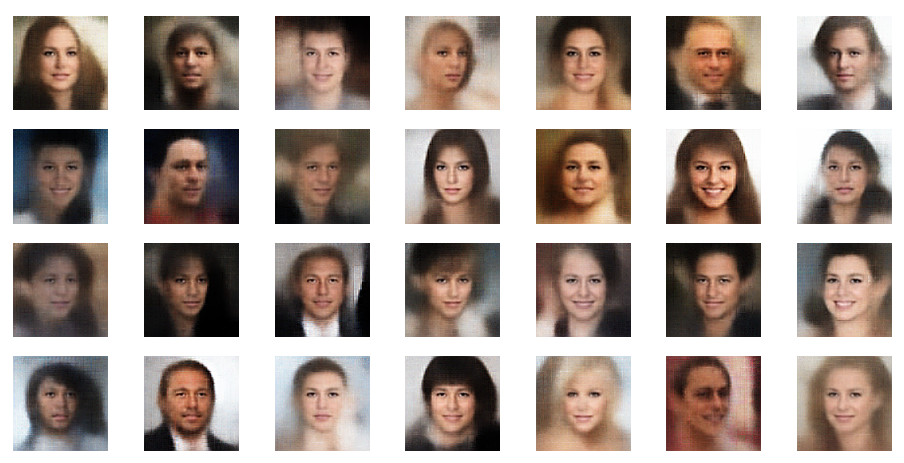Most of these images look quite convincing and crispy down to details. The sharpness results from some photo-processing with PIL functions after the creation by the VAE. But who said that this is not allowed?

# Conclusion

In this post I have presented Jupyter cells with code fragments which may help you to apply the VAE-classes created previously. With the VAE setup discussed above we control the KL-loss by a GradientTape() object.
Preliminary results show that the images created of arbitrarily chosen z-points really show heads with human-like faces and hair-dos. In contrast to what a simple AE would produce (see:
Autoencoders, latent space and the curse of high dimensionality – I

In the next post
Variational Autoencoder with Tensorflow – XI – image creation by a VAE trained on CelebA
I will have a look at the distribution of z-points corresponding to the CelebA data and discuss the delicate balance between the representation of details and the generalization of features. With VAEs you cannot get both.

And let us all who praise freedom not forget:
The worst fascist, war criminal and killer living today is the Putler. He must be isolated at all levels, be denazified and sooner than later be imprisoned. Long live a free and democratic Ukraine!

# Variational Autoencoder with Tensorflow – I – some basics

Last week I tried to perform some systematic calculations with a “Variational Autoencoder” [VAE] for a presentation about Machine Learning [ML]. I wanted to apply VAEs to different standard datasets (MNIST Fashion, CIFAR 10, Celebrity) and demonstrate some basic capacities of VAE algorithms. A standard task … well covered by several introductory books on ML.

Actually, I had some 2 years old Python modules for VAEs available – all based on recommendations in the literature. My VAE-model setup had been done with Keras. Moreprecisely the version integrated into Tensorflow 2 [TF2]. But I ran into severe trouble with my present Tensorflow versions, namely 2.7 and 2.8. The cause of the problems was my handling of the so called Kullback-Leibler [KL] loss in combination with the “eager execution mode” of TF2.

Actually, the problems may already have arisen with earlier TF versions. With this post series I hope to save time for some readers who, as myself, are no ML professionals. In a first post I will briefly repeat some basics about Autoencoders [AEs] and Variational Autoencoders [VAEs]. In a second post we shall build a simple Autoencoder. In a third post I shall turn to VAEs and discuss some variants for dealing with the KL loss – all variants will be picked from recipes of ML teaching books. I shall demonstrate afterward that some of these recipes fail for TF 2.8. A fourth post will discuss the problem with “eager execution” and derive a practical rule for calculations based on layer specific data. In three further posts I apply the rule in the form of three different variants for the KL loss of VAE models. I will set up the VAE models with the help of the functional Keras API. The suggested solutions all do work with Tensorflow 2.7 and 2.8.

If you are not interested in the failures of classical approaches to the KL loss presented in teaching books on ML and if you are not at all interested in theory you may skip the first four posts – and jump to the solutions.

# Some basics of VAEs – a brief introduction

I assume that the reader is already familiar with the concepts of “Variational Autoencoders”. I nevertheless discuss some elements below to get a common vocabulary.

I call the vector space which describes the input samples the "variable space". As AEs and VAEs are typically used for images this “variable space” has many dimensions – from several hundred up to millions. I call the number of these dimensions "n_dim".

An input sample can either be represented as a vector in the variable space or by a multi-ranked tensor. An image with 28×28 pixels and gray “colors” corresponds to a 784 dimensional vector in the variable space or a a “rank 2 tensor” with the shape (28, 28). I use the term “rank” to avoid any notional ambiguities regarding the term “dimensions”. Remember that people sometimes speak of 2-“dimensional” Numpy matrices with 28 elements in each dimension.

An Autoencoder [AE] typically consists of two connected artificial neural networks [ANN]:

• Encoder : maps a point of the “variable space” to a point in the so called “latent space”
• Decoder : maps a point of the “latent space” to a point in the “variable space”.

Both parts of an AE can e.g. be realized with Dense and/or Conv2D layers. See the next post for a simple example of a VAE layer-layout. With Keras both ANNs can be defined as individual “Keras models” which we later connect.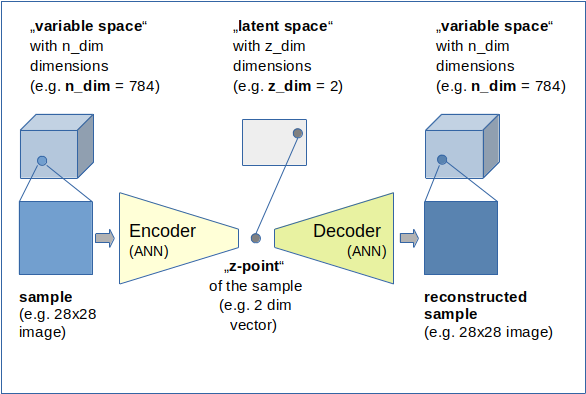Some more details:

• Encoder:
The Encoder ANN creates a vector for each input sample (mostly images) in a low-dimensional vector space, often called the “latent space“. I will sometimes use the abbreviation “z-space” for it and call a point in it a “z-point“. The dimensions of the “z-space” are given by a number “z_dim“. A “z-point” is therefore represented as a z-dimensional vector (a special kind of a tensor of rank 1). The Encoder realizes a non-linear transformation of n-dimensional vectors to z-dimensional vectors. Encoders, actually, are very effective data compressors. z_dim may take numbers between 2 and a few hundred – depending on the complexity of data and objects represented by the input samples with much higher dimensions (some hundreds to millions).
• Decoder:
The “Decoder” ANN instead takes arbitrary low dimensional vectors from the “latent space” as input and creates or better “reconstructs” tensors with the same rank and dimensions as the input samples. These output samples can, of course, also be represented by n-dimensional vectors in the original variable space. The Decoder is a kind of inversion of the “Encoder”. This is also reflected in its structure as we shall see later on. One objective of (V)AEs is that the reconstruction vector for a defined input sample should be pretty close to the input vector of the very same sample – with some metric to measure distances. In natural terms for images: An output image of a Decoder should be pretty comparable to the input image of the Encoder, when we feed the Decode with the z-dim vector created by the Encoder.

A Keras model for the (V)AE can be build by connecting the models for the Encoder and Decoder. The (V)AE model is trained as a whole to minimize differences between the input (e.g. an image) presented to the Encoder and the created output of the Decoder (a reconstructed image). A (V)AE is trained “unsupervised” in the sense that the “label data” for the training are identical to the encoder input samples.

# Encoder and Decoder as CNNs?

When you build the Encoder and the Decoder as a “Convolutional Neutral Networks” [CNNs] they support data compression and reconstruction very effectively by a parallel extraction of basic “features” of the input samples and putting the related information into neural “maps”. (The neurons in such a “map” react sensitively to correlation patterns of components of the tensors representing the input data samples.) For images such CNN networks would accept rank 2 tensors.

Note that the (V)AE’s knowledge about basic “features” (or data correlations) in the input samples is encoded in the weights of the “Encoder” and “Decoder”. The points in the “latent space” which represent the input samples may show cluster patterns. The clusters could e.g. be used in classification tasks. However, without an appropriate Decoder with fitted weights you will not be able to reconstruct a complete image from a point in the z-space. Similar to encryption scenarios the Decoder is a kind of “key” to retrieve original information (even with reduced detail quality).

# Variational Autoencoders

Variational Autoencoders take care about a reasonable data organization in the latent space – in addition to data compression. The objective is to get well defined and segregated clusters there for “features” or maybe even classes of input samples. But the clusters should also be confined in a common, relatively small and compact region of the z-space.

To achieve this goal one judges the arrangement of data points, i.e. their distribution in the latent space, with the help of a special loss contribution. I.e. we use specially designed costs which punish vast extensions of the data distribution off the origin in the latent space.

The data distribution is controlled by parameters, typically a mean value “mu” and a standard deviation or variance “var”. These parameters provide additional degrees of freedom during training. The special loss used to control the predicted distribution in comparison to an ideal one is the so called “Kullback-Leibler” [KL] loss. It measures the “distance” of the real data distribution in the latent space from a more confined ideal one.

You find more information about the KL-loss in the books of D. Foster and R. Atienza named in the last section of this post.

# Why the fuss about VAEs and the KL-loss at all?

Why are VAEs and their “KL loss” important? Basically, because VAEs help you to confine data in the z-space. Especially when the latent space has a very low number of dimensions. VAEs thereby also help to condense clusters which may be dominated by samples of a specific class or a label for some specific feature. Thus VAEs provide the option to define paths between such clusters and allow for “feature arithmetics” via vector operations.

See the following images of MNIST data mapped into a 2-dimensional latent space by a standard Autoencoder: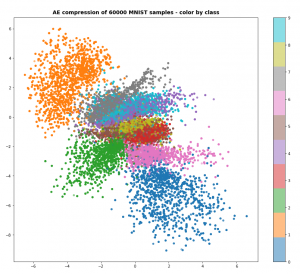Despite the fact that the AE’s sub-nets (CNNs) already deal very well with with features the resulting clusters for the digit classes are spread in a relatively large area of the latent space: [-7 ≤ x ≤ +7], [6 ≥ y ≥ -9]. Had we used dense layers of a MLP only, the spread of data points would have covered an even bigger area in the latent space.

The following three plots resulted from VAEs with different parameters. Watch the scale of the axes.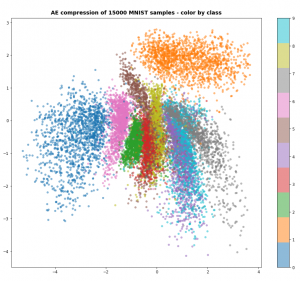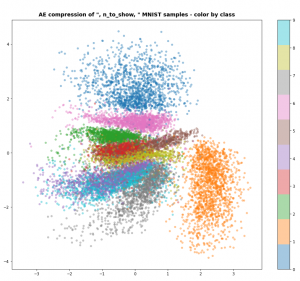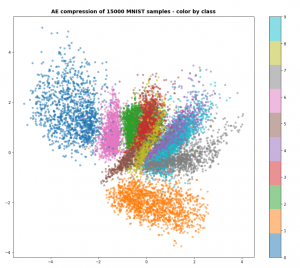The data are now confined to a region of around [[-4,4], [-4,4]] with respect to [x,y]-coordinates in the “latent space”. The available space is more efficiently used. So, you want to avoid any problems with the KL-loss in VAEs due to some peculiar requirements of Tensorflow 2.x.

# Conclusion

AEs are easy to understand, VAEs have subtle additional properties which seem to be valuable for certain tasks. But also for principle reasons we want to enable a Keras model of an ANN to calculate quantities which depend on the elements of one or a few given layers. One example of such a quantity is the KL loss.

In the next post

Variational Autoencoder with Tensorflow 2.8 – II – an Autoencoder with binary-crossentropy loss

we shall build an Autoencoder and apply it to the MNIST dataset. This will give us a reference point for VAEs later.

Stay tuned ….
Ceterum censeo: The worst living fascist and war criminal today, who must be isolated, denazified and imprisoned, is the Putler.